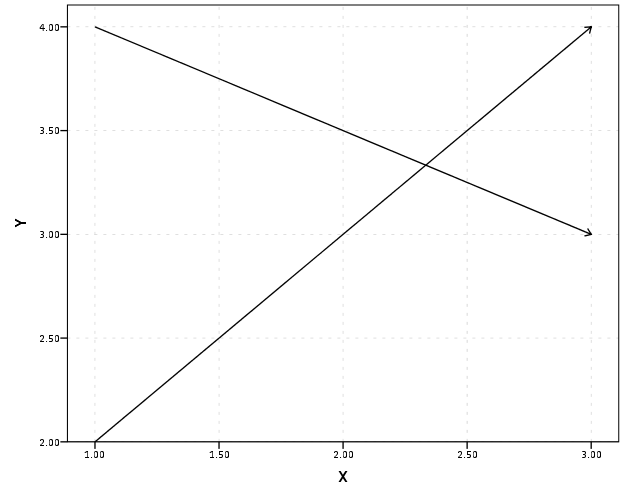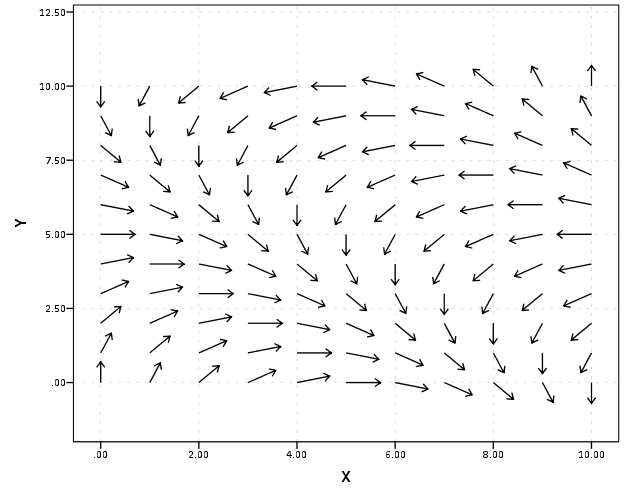# Using the edge element in SPSS inline GPL with arbitrary X,Y coordinates

This post is intended to be a brief illustration of how one can utilize the edge element in SPSS’s GGRAPH statements to produce vector flow fields. I post this because the last time I consulted the GGRAPH manual (which admittedly is probably a few versions olds) they only have examples of SPSS’s ability to utilize random graph layouts for edge elements. Here I will show how to specify the algebra for edge elements if you already have X and Y coordinates for the beginning and end points for the edges. Also to note if you want arrow heads for line elements in your plots you need to utilize edge elements (the main original motivation for me to figure this out!)

So here is a simple example of utilizing coordinate begin and end points to specify arrows in a graph, and below the code is the image it produces.

``````DATA LIST FREE/ X1 X2 Y1 Y2 ID.
BEGIN DATA
1 3 2 4 1
1 3 4 3 2
END DATA.

GGRAPH
/GRAPHDATASET NAME="graphdataset" VARIABLES=X1 Y1 X2 Y2 ID MISSING=LISTWISE REPORTMISSING=NO
/GRAPHSPEC SOURCE=INLINE.
BEGIN GPL
SOURCE: s=userSource(id("graphdataset"))
DATA: X1=col(source(s), name("X1"))
DATA: Y1=col(source(s), name("Y1"))
DATA: X2=col(source(s), name("X2"))
DATA: Y2=col(source(s), name("Y2"))
DATA: ID=col(source(s), name("ID"))
GUIDE: axis(dim(1), label("X"))
GUIDE: axis(dim(2), label("Y"))
ELEMENT: edge(position((X1*Y1)+(X2*Y2)), shape(shape.arrow))
END GPL.``````The magic happens in the call to the edge element, specifically the graph algebra position statement of `(X1*Y1)+(X2*Y2)`. I haven’t read Wilkinson’s Grammar of Graphics, and I will admit with SPSS’s tutorial on graph algebra at the intro to the GPL manual it isn’t clear to me why this works. I believe the best answer I can say is that different elements have different styles to specify coordinates in the graph. For instance interval elements (e.g. bar charts) can take a location in one dimension and an interval in another dimension in the form of `X*(Y1+Y2)` (see an example in this post of mine on Nabble – I also just found in my notes an example of specifying an edge element in the similar manner to the interval element). This just happens to be a valid form to specify coordinates in edge elements when you aren’t using one of SPSS’s automatic graph layout rendering. I guess it is generically of the form `FromNode(X*Y),ToNode(X*Y)`, but I haven’t seen any documentation for this and all of the examples I had seen in the reference guide utilize a different set of nodes and edges, and then specify a specific type of graph layout.

Here is another example visualizing a vector flow field. Eventually I would like to be able to superimpose such elements on a map – but that appears to not yet be possible in SPSS.

``````set seed = 10.
input program.
loop #i = 0 to 10.
loop #j = 0 to 10.
compute X = #i.
compute Y = #j.
*compute or_deg = RV.UNIFORM(0,360).
end case.
end loop.
end loop.
end file.
end input program.
dataset name sim.
execute.

*making orientation vary directly with X & Y attributes.

compute or_deg = 18*X + 18*Y.

*now to make the edge I would figure out the X & Y coordinate with alittle distance added (lets say .01) based on the orientation.
COMPUTE #pi=4*ARTAN(1).
compute distance = .7.
execute.

compute X2 = X + sin(or_rad)*distance.
compute Y2 = Y + cos(or_rad)*distance.
execute.

DATASET ACTIVATE sim.
* Chart Builder.
GGRAPH
/GRAPHDATASET NAME="graphdataset" VARIABLES=X Y X2 Y2 or_deg MISSING=LISTWISE REPORTMISSING=NO
/GRAPHSPEC SOURCE=INLINE.
BEGIN GPL
SOURCE: s=userSource(id("graphdataset"))
DATA: X=col(source(s), name("X"))
DATA: Y=col(source(s), name("Y"))
DATA: X2=col(source(s), name("X2"))
DATA: Y2=col(source(s), name("Y2"))
DATA: or_deg=col(source(s), name("or_deg"))
GUIDE: axis(dim(1), label("X"))
GUIDE: axis(dim(2), label("Y"))
ELEMENT: edge(position((X*Y)+(X2*Y2)), shape(shape.arrow))
END GPL.``````You can then use other aesthetics in these charts same as usual (color, size, transparency, etc.)